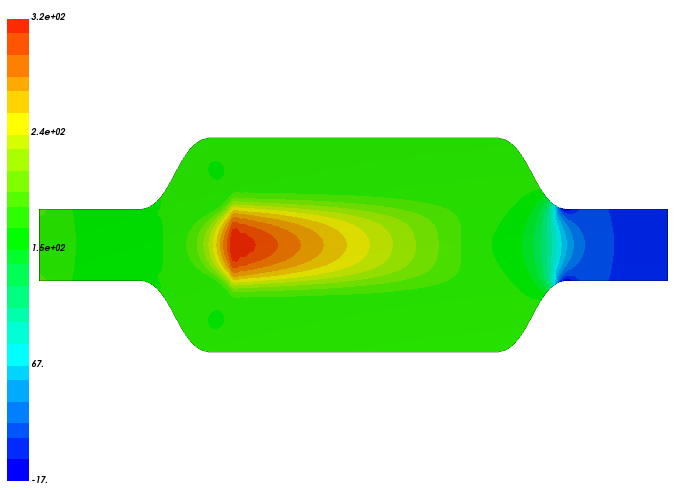Back to all tutorials

# Catalytic Converter - CFD Simulation SimFlow Tutorial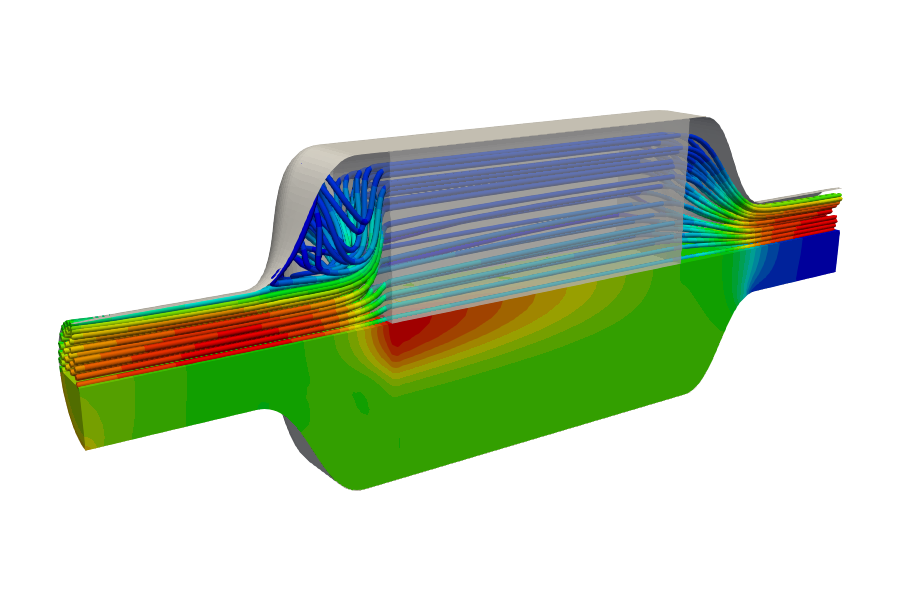## 1. Introduction

In this tutorial, we will be demonstrating how to model a porous medium. We have simulated the flow through the catalytic converter using the Darcy-Forchheimer porosity model. The inlet velocity has been described by a formula that depends on the coordinates. Second-order velocity discretization (Linear Upwind) has been used to increase the accuracy of the simulation.

SimFlow is a general purpose CFD Software

## 3. Create Case

Open SimFlow and create a new case named catalytic_converter

1. Go to panel
2. Provide name catalytic_converter
3. Click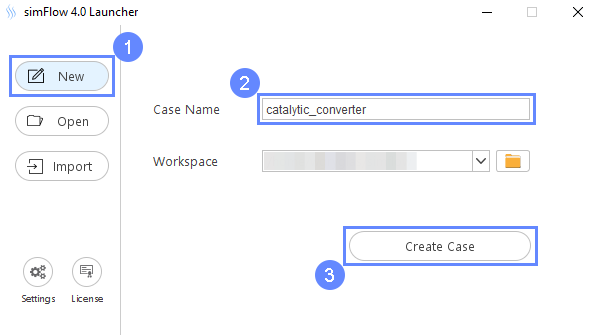## 4. Import Geometry

1. Click
2. Select geometry file catalytic-converter.stl
3. Click to import geometry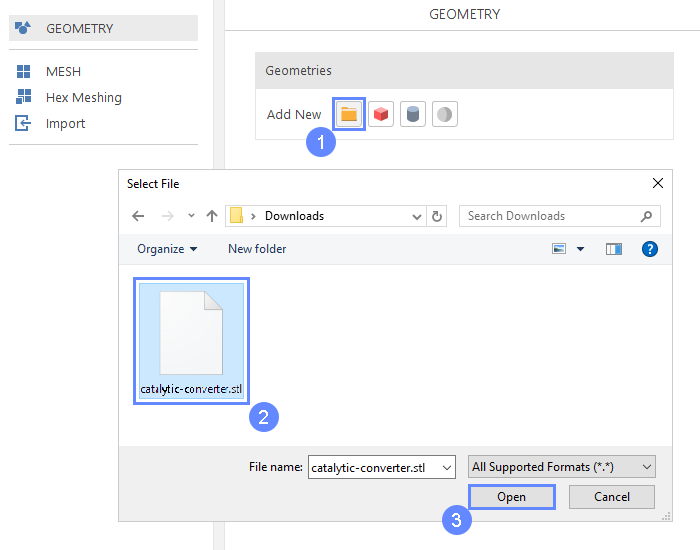## 5. Imported Geometry Units

Since the imported geometry is in STL format, which does not store unit information, we need to confirm the unit in which the model was created. In the selection of unit, we can use the Geometry size label, which displays overall size of the model in each direction. In our case, the model was created in millimeters.

1. Select mm unit
2. Press button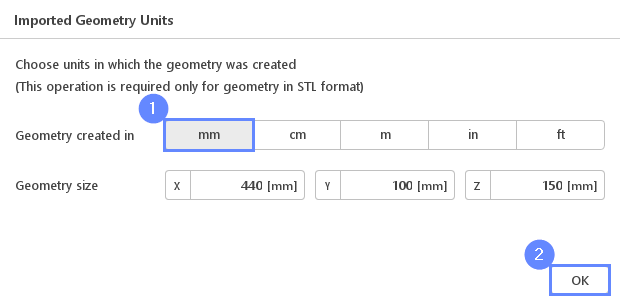## 6. Geometry - Catalytic Converter

After importing geometry, it will appear in the 3D window.

1. Click to zoom in on the geometry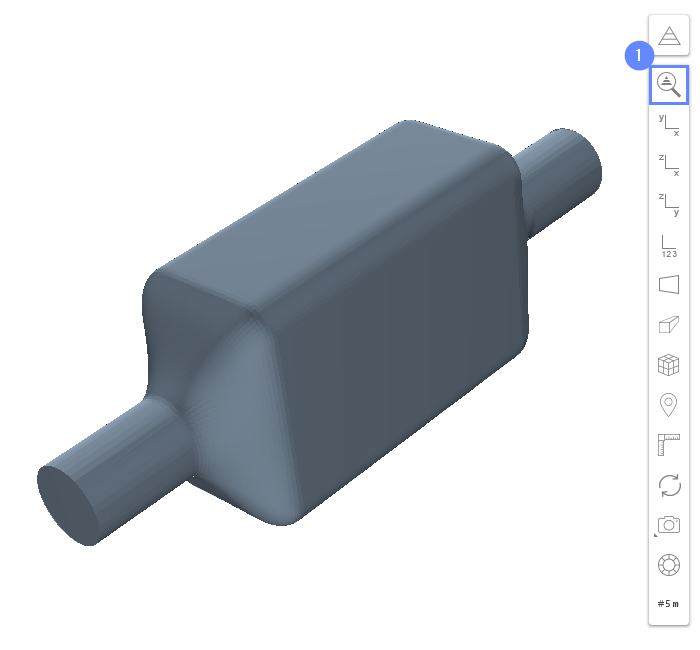## 7. Split Geometry - Catalytic Converter (I)

The imported geometry is made up of a single surface. We need to split it into multiple faces for further processing.

1. Expand the Options list for catalytic_converter
2. Select Split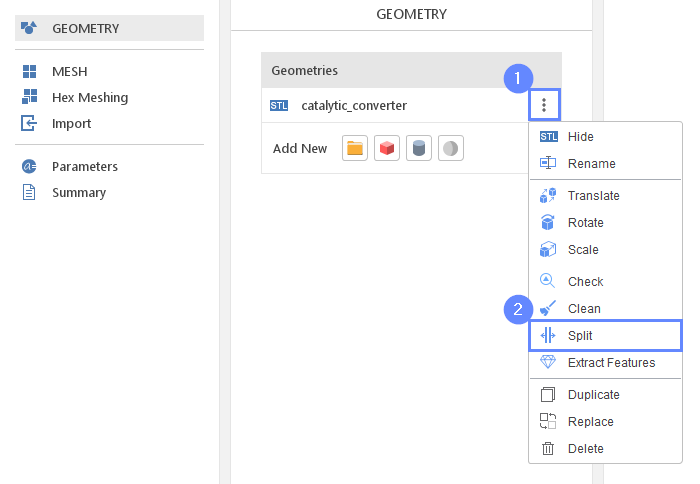## 8. Split Geometry - Catalytic Converter (II)

In order to split original geometry into separate objects, we will disable Create Single Geometry options:

1. Uncheck Create Single Geometry
2. Click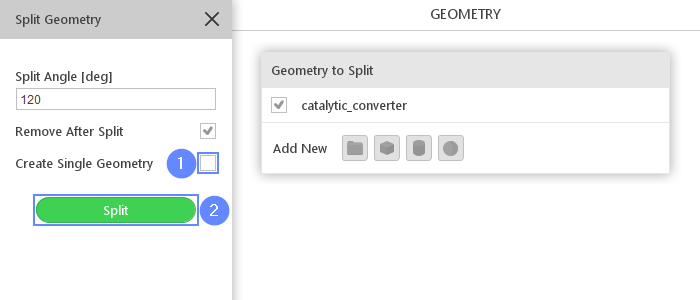## 9. Rename Geometries

To make the resulting mesh boundaries more readable we will rename each geometry face:

1. Select catalytic_converter_patch00 from the list. The selected face will be highlighted in the 3D graphic window
2. Double click on the name and change it to match the picture below
catalytic_converter_patch00 $$\rightarrow$$ inlet
3. Repeat these steps for the remaining geometry components
catalytic_converter_patch01 $$\rightarrow$$ outlet
catalytic_converter_patch02 $$\rightarrow$$ wall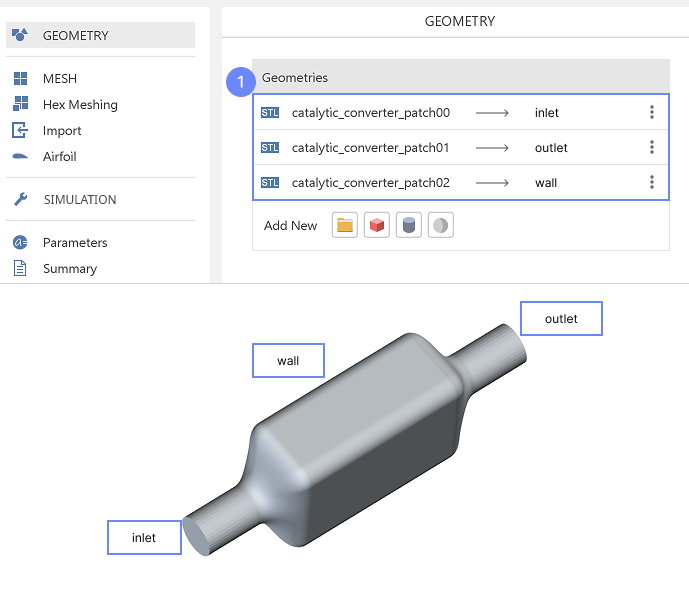## 10. Create Geometry - Cell Zone

In this tutorial, we would like to model a porous zone inside the catalytic converter. To do so we need to define the region where the porous resistance will be applied. For this purpose we will create new box geometry:

1. Click on Create Box
2. Set the origin and box dimensions accordingly
Origin $${\sf [m]}$$-0.085-0.06-0.09
Dimensions $${\sf [m]}$$0.1750.120.18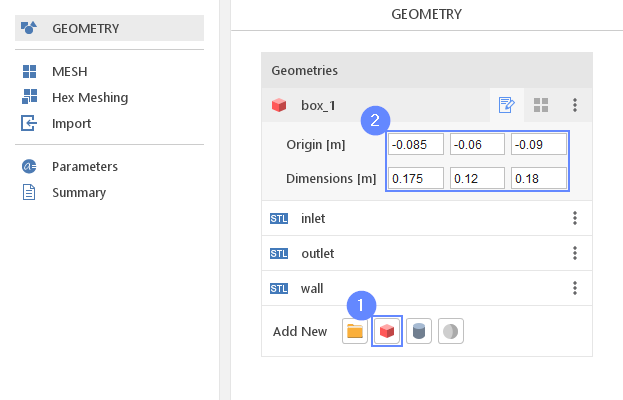## 11. Meshing Properties - Catalyctic Converter

In order to create the mesh, we need to specify geometries options used during the meshing process.

1. Go to Hex Meshing panel
2. Enable Mesh Geometry on the inlet outlet wall
3. Enable Create Boundary Layer Mesh on wall geometry
4. Click on the wall in the listto expand options
5. Set No. Of Layers to 4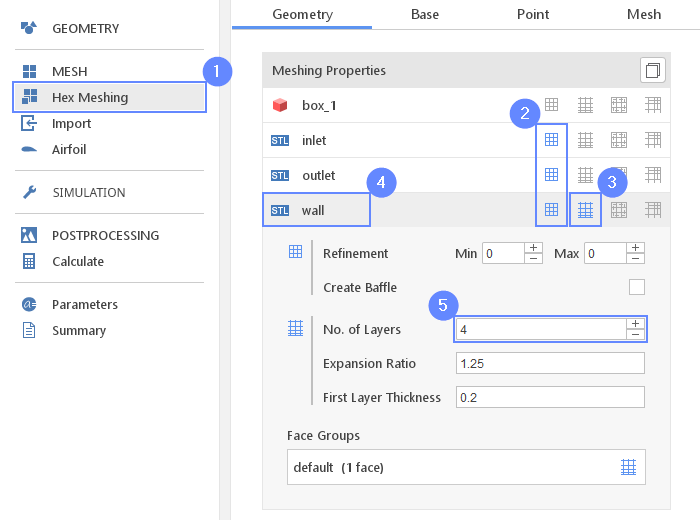## 12. Base Mesh - Domain

Now we will define the base mesh. The box geometry determines the background mesh domain. The model is symmetrical on the XZ plane, so we do not need to mesh the whole geometry. Using the box dimensions we will choose only half of the domain.

1. Go to Base tab
2. Click on
3. Change Max [m] Y to 0
4. Define the number of divisions
Division1551750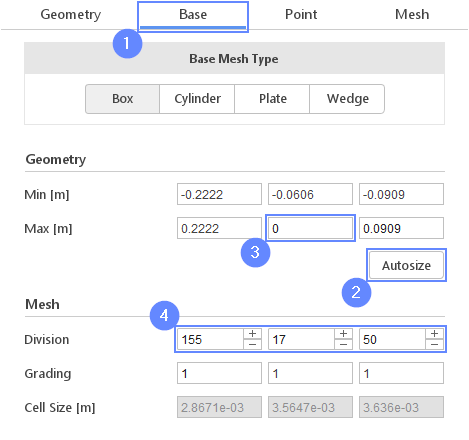## 13. Material Point

Material Point tells the meshing algorithm on which side of the geometry the mesh is to be retained. Since we are considering flow inside the catalytic converter we need to place the material point inside the geometry.

1. Go to Point tab
2. Specify location inside the catalytic converter geometry
Material Point0-0.020

You can specify the point location from the 3D view. Hold the CTRL key and drag the arrows to the destination.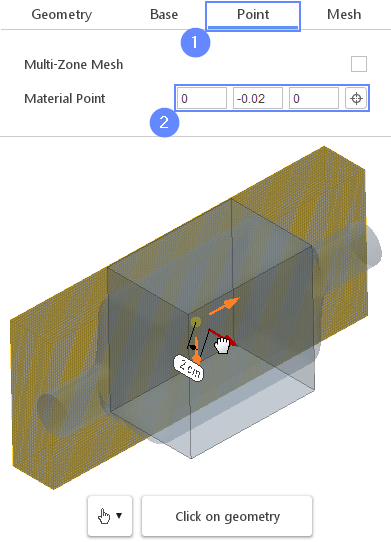## 14. Start Meshing

Everything is now set up for meshing

1. Go to Mesh tab
2. Start the meshing process with button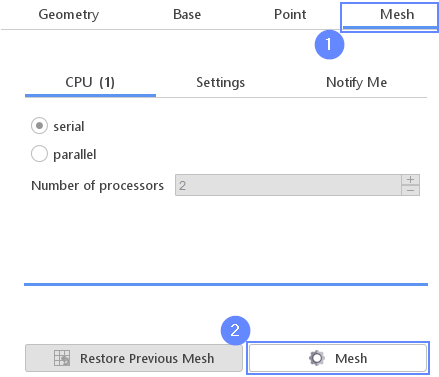## 15. Mesh

After the meshing process is finished the mesh will appear in the graphics window.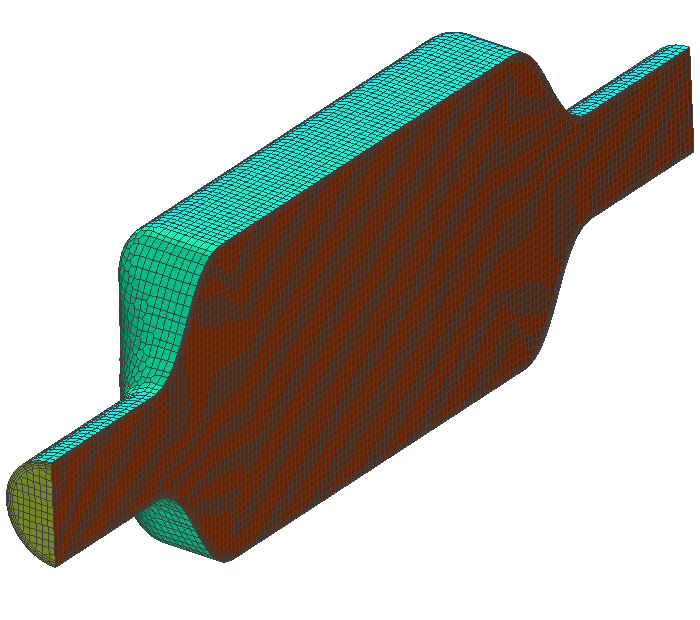## 16. Create Cell Zone (I)

Now we will define the cell zone using box_1 geometry.

1. Expand the Options list next to default region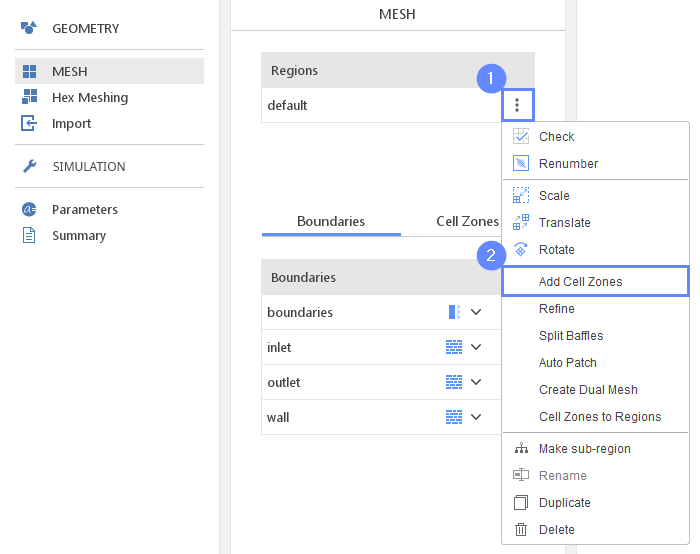## 17. Create Cell Zone (II)

1. Check the box_1
2. Click on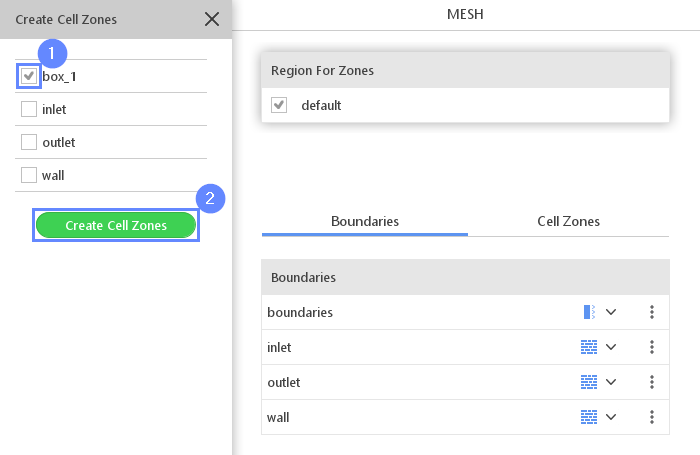## 18. Display Cell Zone

The cell zone appears inside the catalytic converter. It is colored on light blue.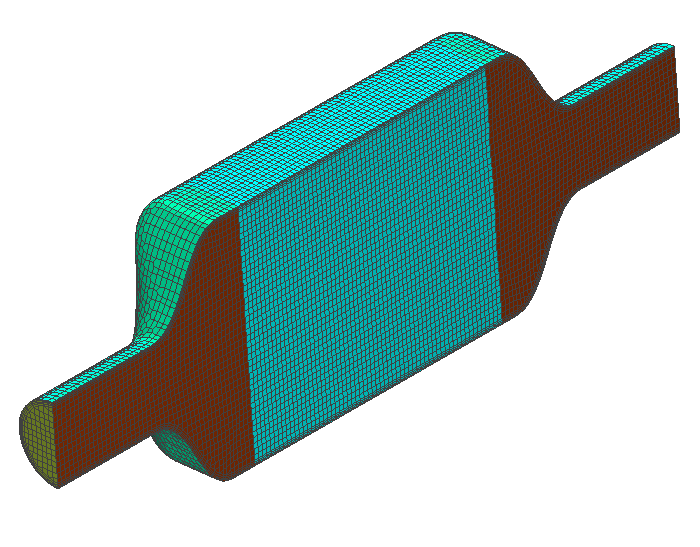## 19. Boundaries

1. Change boundary name from boundaries $$\rightarrow$$ symmetry
2. Define boundary types accordingly
inlet Patch
outlet Patch
symmetry Symmetry
wall Wall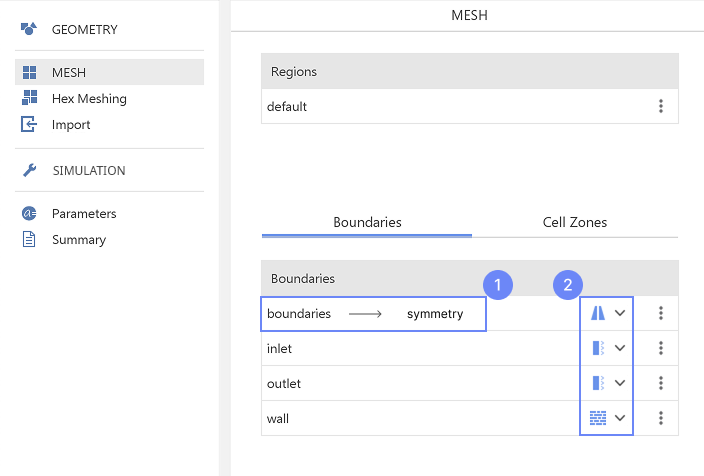## 20. Select Solver - SIMPLE

We want to analyze internal, incompressible turbulent flow. For this purpose, we will use the SIMPLE (simpleFoam) solver.

1. Go to SETUP panel
2. Enable filter
3. Filter the solvers by flow
4. Pick SIMPLE (simpleFoam) solver
5. solver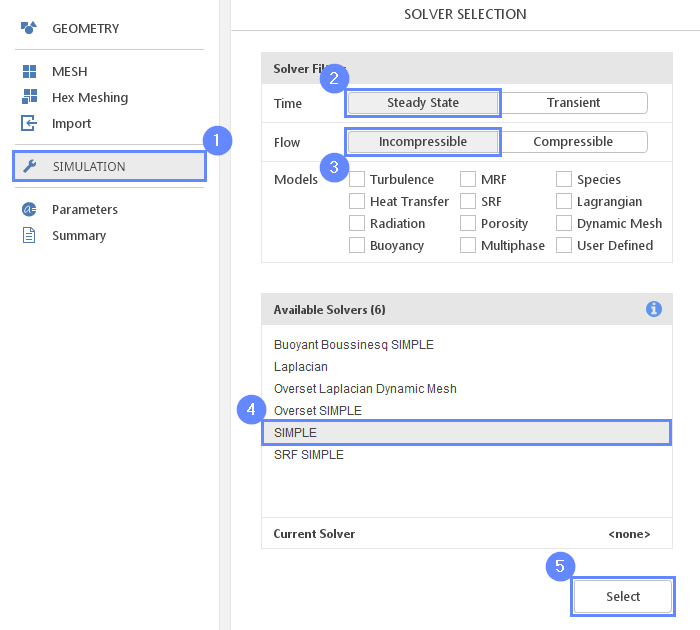## 21. Turbulence

For turbulence modeling, we will use the standard $$k-\varepsilon$$ model.

1. Go to Turbulence panel
2. Select RANS for Turbulence Modeling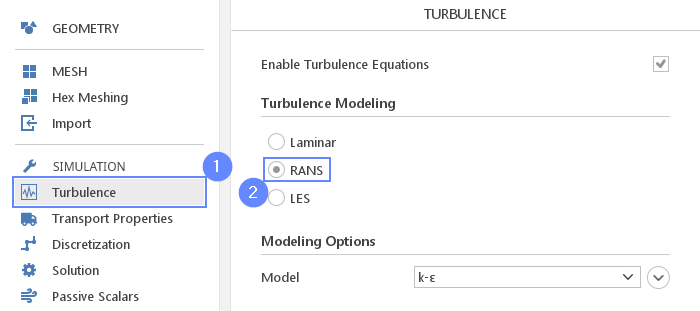## 22. Transport Properties

We will change the kinematic viscosity to the value of the exhaust gas.

1. Go to Transport Properties panel
2. Set the kinematic viscosity
$$\nu$$ $${\sf [\frac{m^2}{s}]}$$1.272e-04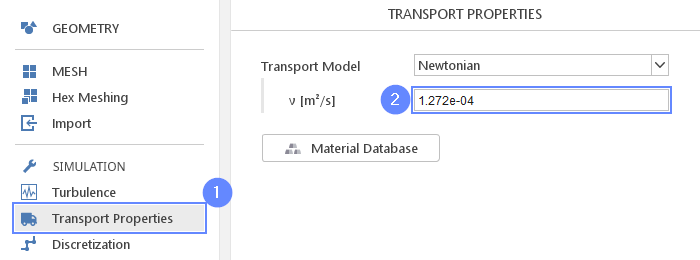## 23. Cell Zones - Porosity

In order to include porosity to our simulation we have to define porosity for the box_1 cell zone.

1. Go to Cell Cones panel
2. Select the box_1 zone
3. Check Porous Zone
4. Use default Darcy-Forchheimer model and set its parameters accordingly
d $${\sf [\frac{1}{m^2}]}$$3e-073e-103e-10
f $${\sf [\frac{1}{m}]}$$202000020000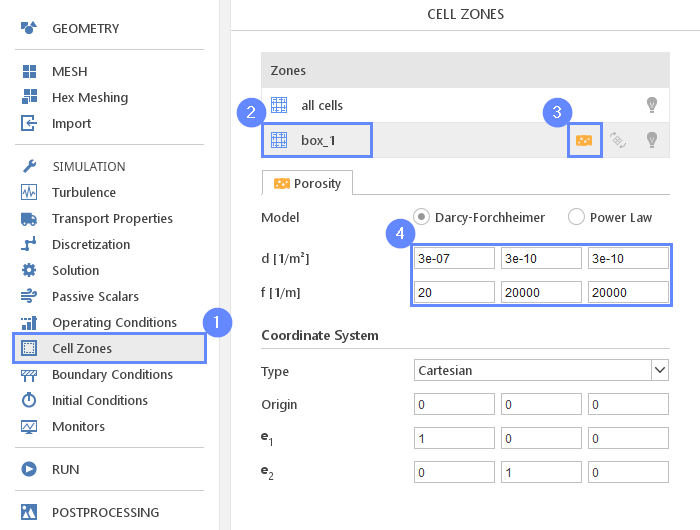## 24. Parameters - R, U

SimFlow allows users to create named parameters that can be used instead of numbers. This allows to easily change a single property without the need to edit all inputs where a given property is used. In this tutorial, we will define two global parameters that we will use during further setup.

1. Go to Parameters panel
2. Definethe new parameter
Name R Formula 0.025
3. Click Create Parameter
4. Repeat these steps for the second parameter
Name U Formula 20
The newly created parameters will be shown in the parameters list.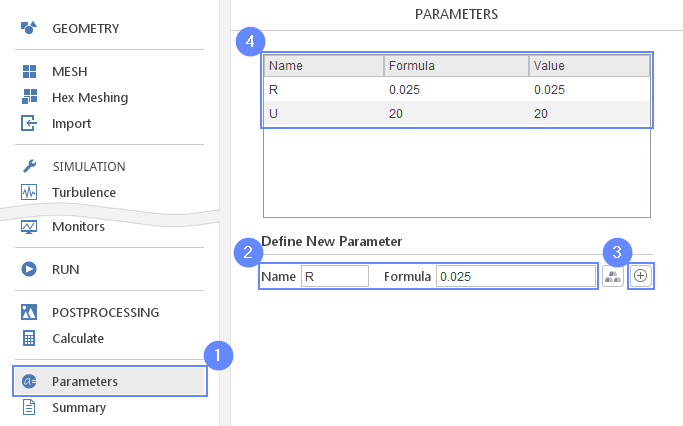## 25. Boundary Conditions - Inlet (Flow)

We will set inlet velocity as a parabolic profile. The profile we will define by the formula using U and R parameters. Velocity magnitude near the wall will be equal to zero and the highest value of U will be in the center of the pipe.

1. Go to Boundary Conditions panel
2. Select the inlet boundary
3. Set the Velocity Inlet character
4. Change the velocity type and value accordingly
U Type Fixed Value
U Value $${\sf [\frac{m}{s}]}$$U*( (R-sqrt(y^2 +z^2 ))/R)^(1/7)00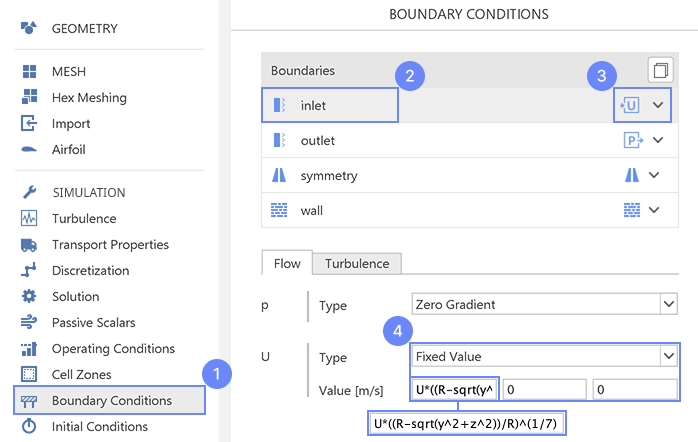## 26. Save Case

To visualize and check velocity distribution we will open ParaView. Firstly we will save the case.

1. Go to RUN panel
2. Press Save Case button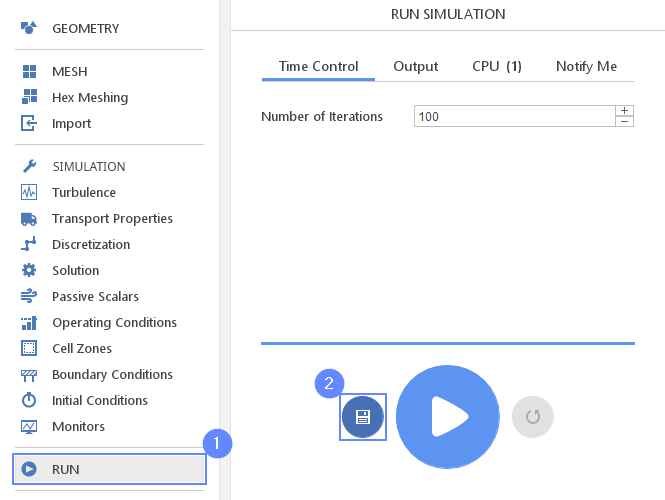## 27. Check Velocity Profile - ParaView (I)

Run the ParaView from the Postprocessing panel.

1. Go to POSTPROCESSING panel
2. Click on Run ParaView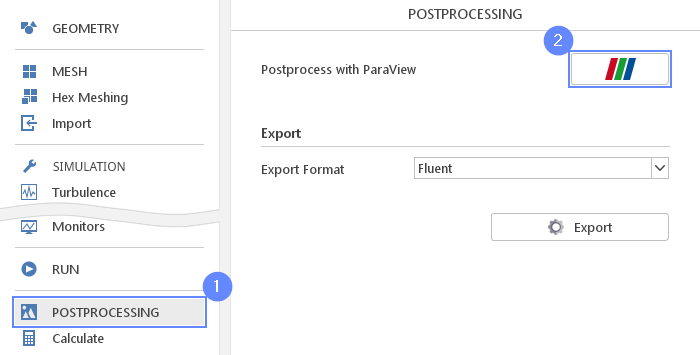## 28. Check Velocity Profile - ParaView (II)

We will display a velocity magnitude map on the inlet.

1. Make sure that catalytic_converter is selected
2. Press
3. Uncheck Skip Zero Time
4. Press
5. Press
6. Select velocity U from the list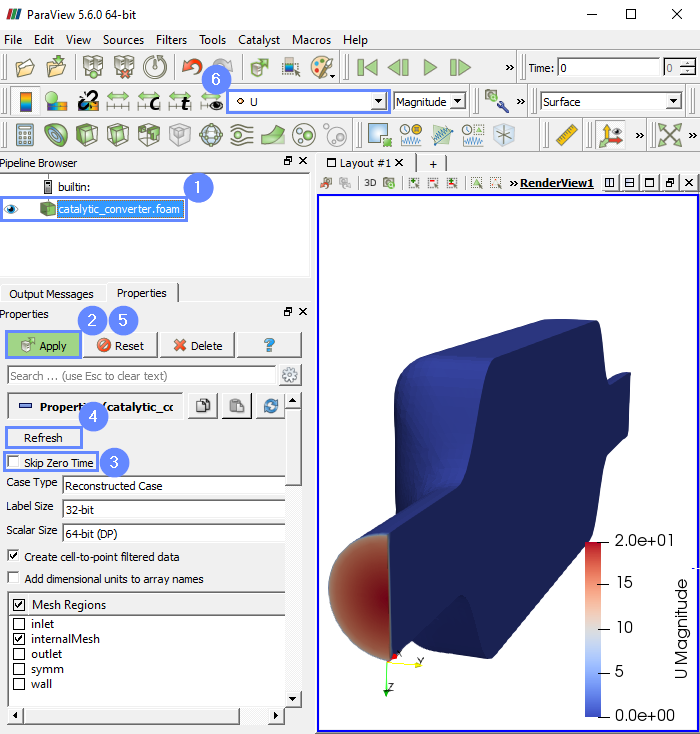## 29. Boundary Conditions - Inlet (Turbulence)

Back in SimFlow, we will also update the turbulent mixing length for the ε-equation at the inlet.

1. Go to Boundary Conditions panel
2. Select inlet boundary
3. Switch to Turbulence tab
4. Set the value of Mixing Length to 7% of inlet diameter
$$\varepsilon$$ Mixing Length $${\sf [m]}$$0.07*2*R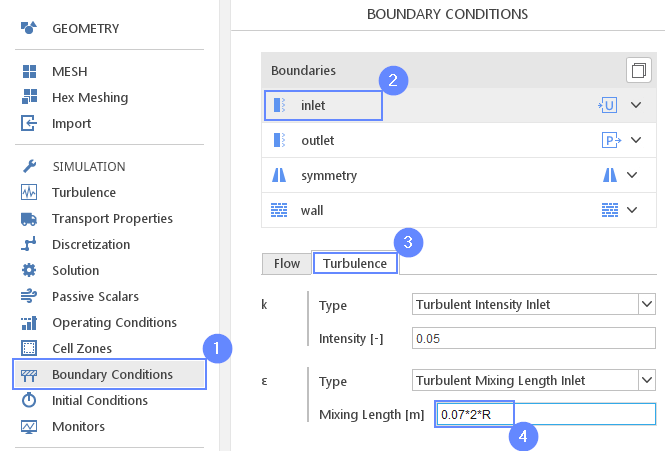## 30. Initial Conditions - Potential

In order to give a better initial guess for velocity and pressure fields, we will use the "Potential" initialization feature. This utility solves pseudo potential flow prior to actual calculations.

1. Go to Initial Conditions panel
2. Switch to Potential tab
3. Check the Initialize Potential Flow
4. Set No. of correctors to 10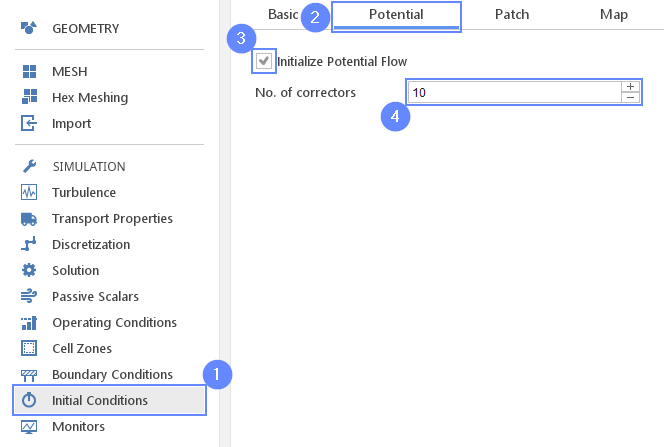## 31. Monitors - Boundary

We would like to monitor how results change during computation. We will enable plotting the average pressure at the inlet during the calculation:

1. Go to Monitors panel
2. Switch to Boundary tab
3. Expand Fields list next to inlet boundary
4. Check the pressure p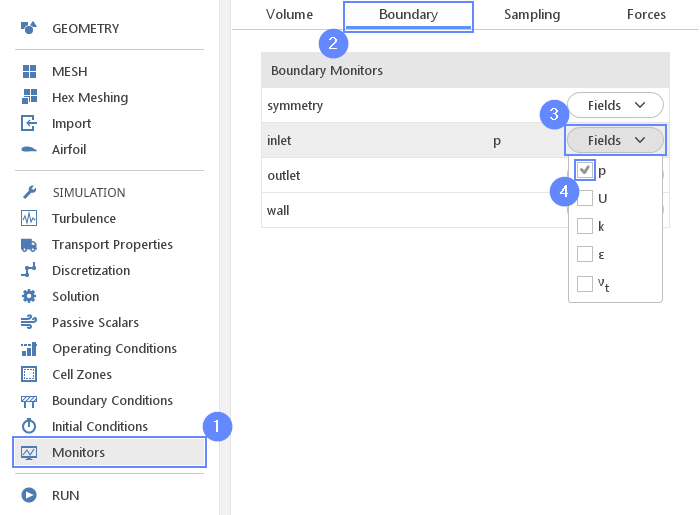## 32. Monitors - Sampling

We will also display intermediate results on a section plane.

1. Go to Sampling tab
3. Set the normal vector
Normal $${\sf [-]}$$010
5. Choose the pressure p and the velocity U to be sampled on the section plane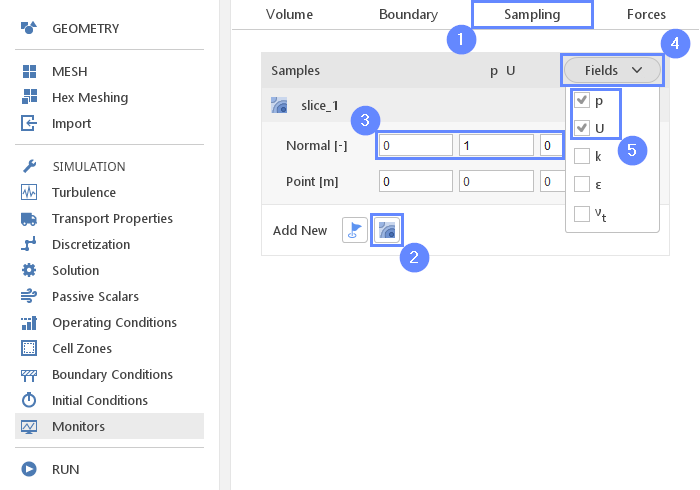## 33. Run - Time Control

1. Go to RUN panel
2. Set the maximal Numbers of Iterations to 300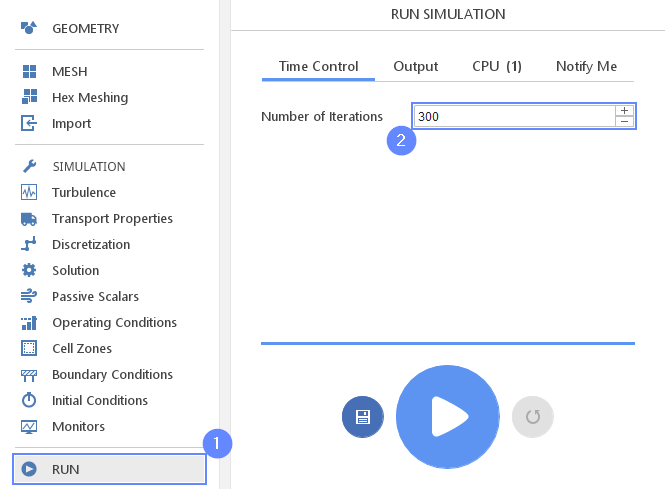## 34. Run - Output

In this tutorial, we run a steady-state simulation. We are only interested in the latest time step. However, it is advised to keep the previous time step result as a backup on the disk. We will make a backup every 50 iterations.

1. Switch to Output tab
2. Set the Interval [-] to 50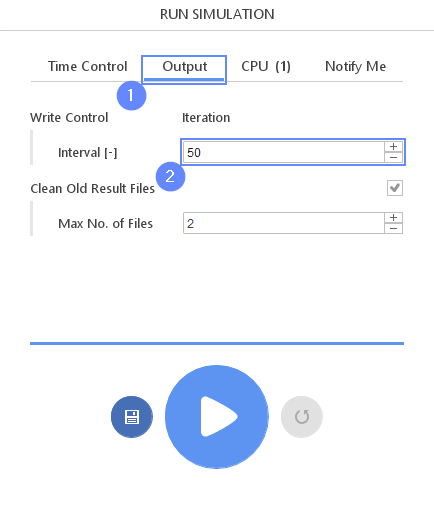## 35. Run - CPU

To speed up the calculation process increase the number of CPUs basing on your PC capability. The free version allows you to use only 2 processors in parallel mode. To get the full version, you can use the contact form to Request 30-day Trial

Estimated computation time for 2 processors: 5 minutes

1. Switch to CPU tab
2. Use parallel mode
3. Increase the Number of processors
4. Click button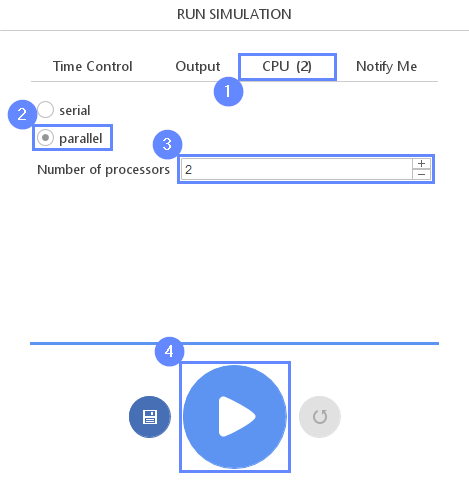## 36. Control Inlet Pressure

Go to the p on inlet tab to observe convergence process.

1. Switch to p on inlet tab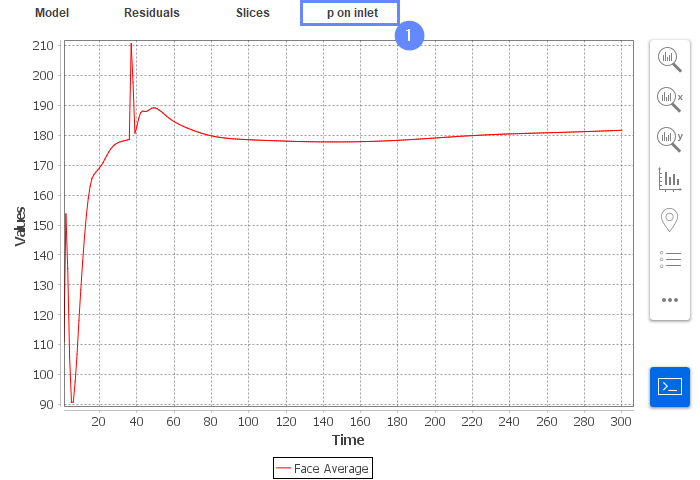## 37. Discretization

After preliminary calculation, we will continue with a more accurate algorithm. Change velocity discretization to the Linear Upwind scheme to minimize numerical diffusion affecting results of the simulation.

1. Go to Discretization panel
2. Switch to Convection tab
3. Click on to extend the list
4. Select Linear Upwind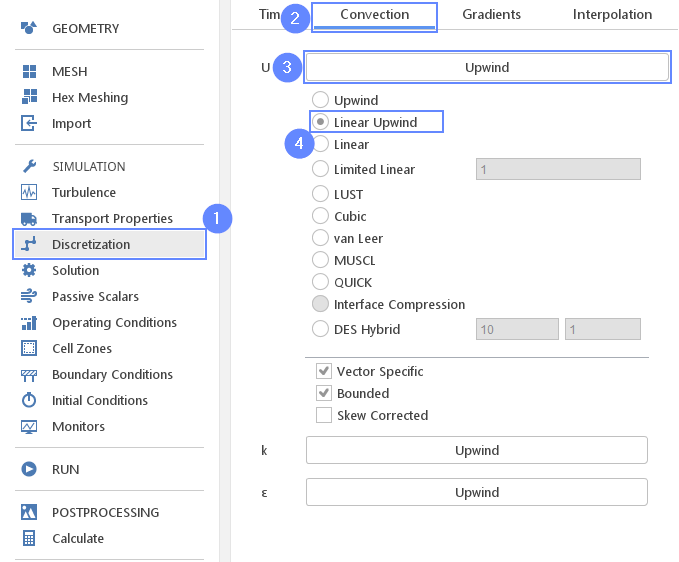## 38. Run - Continue Simulation

1. Go to RUN panel
2. Set the maximal Numbers of Iterations to 2000
3. Click button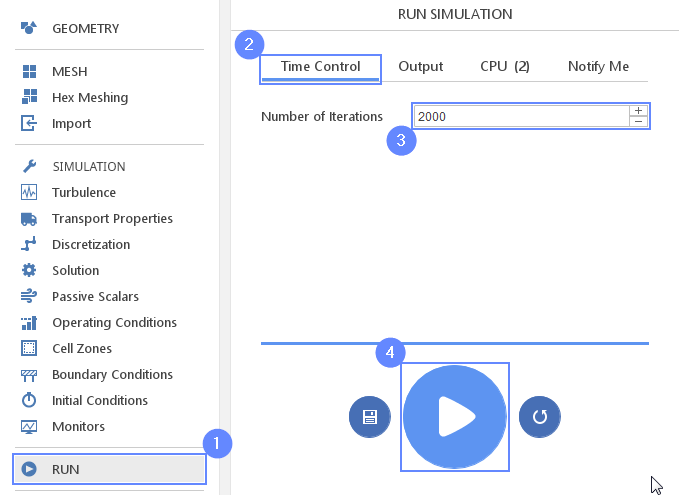## 39. Control Inlet Pressure - New Scheme

Go back to the p on inlet tab and observe convergence process.

1. Switch to p on inlet tab
2. Control the convergence of the pressure on the inlet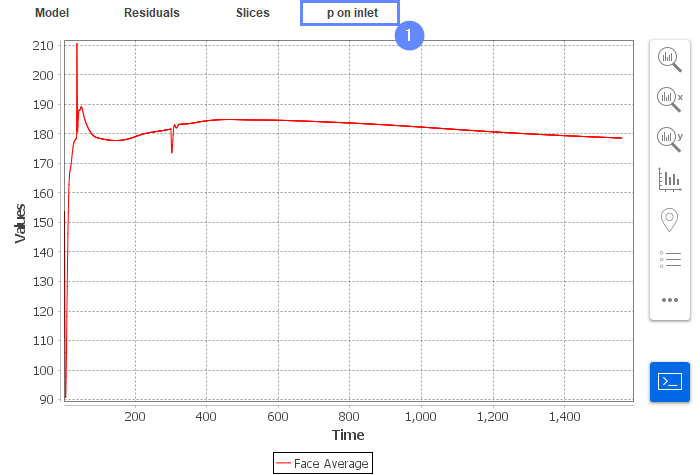## 40. Slice - View Velocity Field

Slices tab appears next to the Residuals tab. Under this tab, we can preview results on the defined section plane.

1. Change tab to Slices
2. Select the velocity U
3. Click Adjust range to data
4. Click
5. Set the XZ orientation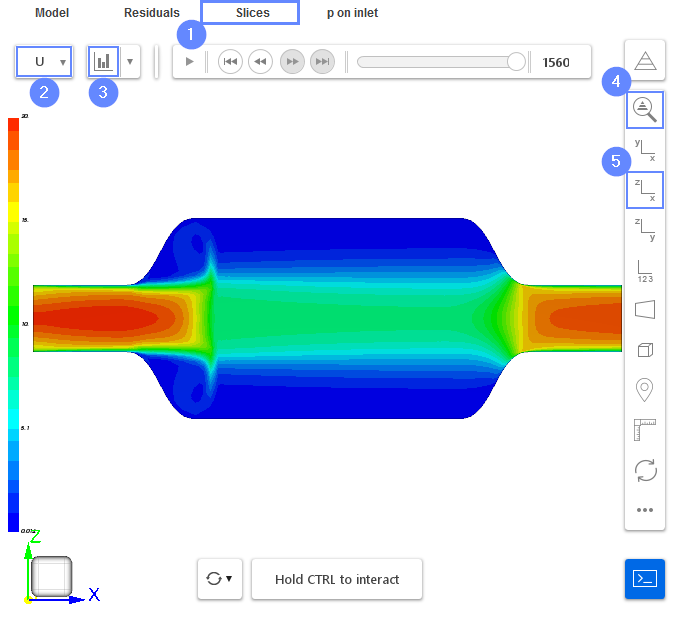## 41. Slice - Final Results

At the end, we will look at the pressure distribution. The Darcy porous model assumes resistance and hence pressure gradient to be proportional to the velocity in the porous zone. We can observe the effect of increased resistance from the porous zone. The pressure builds up in front of the porous zone and then linearly decreases along the flow direction due to resistance.